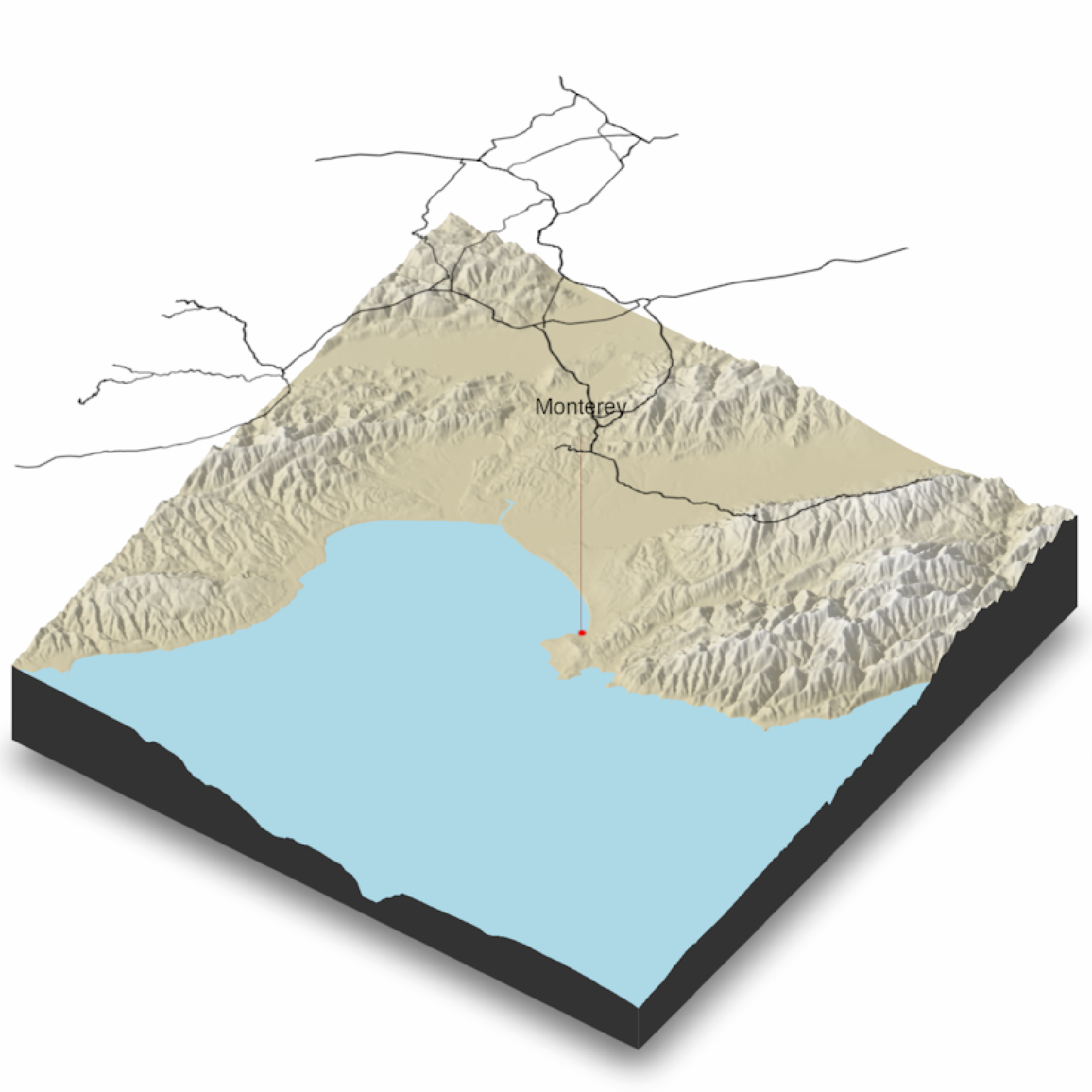Render a 2D floating overlay over the map.

Note: Multiple layers with transparency can cause rendering issues in rgl.

render_floating_overlay(
overlay = NULL,
altitude = NULL,
heightmap = NULL,
zscale = 1,
alpha = 1,
baseshape = "rectangle",
remove_na = TRUE,
reorient = TRUE,
clear_layers = FALSE,
horizontal_offset = c(0, 0),
...
)

## Arguments

overlay

Overlay (4D RGBA array) to be rendered on the 3D map.

altitude

Altitude to place the overlay.

heightmap

The underlying surface. A two-dimensional matrix, where each entry in the matrix is the elevation at that point.

zscale

Default 1. The ratio between the x and y spacing (which are assumed to be equal) and the z axis. For example, if the elevation levels are in units of 1 meter and the grid values are separated by 10 meters, zscale would be 10. Adjust the zscale down to exaggerate elevation features.

alpha

Default 1. Multiplies the layer's transparency by this factor. 0 is completely transparent.

baseshape

Default rectangle. Shape of the overlay. Options are c("rectangle", "circle", "hex").

remove_na

Default TRUE. Whether to make the overlay transparent above empty spaces (represented by NA values) in the underlying elevation matrix.

reorient

Default TRUE. Whether to reorient the image array to match the 3D plot.

clear_layers

Default FALSE. Clears all existing floating layers on the visualization.

horizontal_offset

Default c(0,0). Distance (in 3D space) to offset the floating offset in the x/y directions.

...

Additional arguments to pass to rgl::triangles3d().

## Value

Adds a 3D floating layer to the map. No return value.

## Examples

if(rayshader:::run_documentation()) {
#Render the road network as a floating overlay layer, along with a label annotation and a floating
#point annotation
if(all(length(find.package("sf", quiet = TRUE)) > 0,
length(find.package("magick", quiet = TRUE)) > 0)) {
monterey = c(-121.892933,36.603053)
monterey_city = sf::st_sfc(sf::st_point(monterey))

#Generate Overlays
heightmap = montereybay)
point_overlay = generate_point_overlay(monterey_city, color="red", size=12,
attr(montereybay,"extent"), heightmap = montereybay)

#Create 3D plot (water transparency set to 1 because multiple transparency layers can interfere)
montereybay |>
plot_3d(montereybay, water = T, wateralpha = 1, windowsize = 800, watercolor = "lightblue")
render_camera(theta=-55,phi=45,zoom=0.8)

#Render label
render_label(montereybay, lat = monterey, long = monterey, altitude = 9900,
extent = attr(montereybay, "extent"),
zscale = 50, text = "Monterey", textcolor = "black", linecolor="darkred")

#Render Floating Overlays
render_floating_overlay(road_overlay, altitude = 10000,zscale = 50)
render_floating_overlay(point_overlay, altitude = 100,zscale = 50)
render_snapshot()
}
}
#> montereybay dataset used with no zscale--setting zscale=50.  For a realistic depiction, raise zscale to 200.